# A class IV.A

In a class there are 15 girls and 30 boys.  What fraction of the class represents the boys?

x =  0.6667

### Step-by-step explanation:Did you find an error or inaccuracy? Feel free to write us. Thank you!Tips to related online calculators
Need help to calculate sum, simplify or multiply fractions? Try our fraction calculator.

## Related math problems and questions:

• A class IV.CIn a class 2/5 were boys. 30 were girls. How many more girls than boys are there?
• Ratio of countsThere are 15 boys and 13 girls in the class. What are the ratio of boys and girls?
• Prospering studentsThere are girls and 30 boys in the class. Boys prospering 28, girls all. How many girls are in the class and how many boys are there, if all the prospering students are 95%?
• The ratioThe ratio of girls to boys in Mrs. White's class is 3:2.  If there are 12 boys, how many girls are in the class room?
• There 12There are 42 students in the class and 2/3 of them are girls. How may girls are there in the class?
• Children 9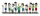There are 11 children in a room. 6 of the children are girls. What fraction of the children are girls?
• Classroom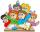In a class are 32 pupils. Boys are 8 less than girls. How many boys and girls are in the classroom?
• Pupils 7There are 40 pupils in a certain class. 3/5 of the class are boys. How many are girls?
• Classroom 4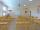In a class of 36 pupils, 2/3 are girls. How much it is in a class girls and boys?
• Simplest form 3What fraction is 15 of 35 in simplest form?
• PupilsThere are 32 pupils in the classroom, and girls are two-thirds more than boys. a) How many percents are more girls than boys? Round the result to a whole percentage. b) How many boys are in the class? c) Find the ratio of boys and girls in the class. Writ
• Boys and girlsThere are eight boys and nine girls in the class. There were six children on the trip from this class. What is the probability that left a) only boys b) just two boys
• GroupsIn the 6th class there are 60 girls and 72 boys. We want to divide them into groups so that the number of girls and boys is the same. How many groups can you create? How many girls will be in the group?
• In the classroomThere are 30 boys and a few girls in the class. In the six months, 28 boys and all girls benefited, which was 95% of all pupils. How many pupils are there in the classroom?
• Glasses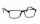There are 36 pupils in the class. Nine girls wear glasses. Boys with glasses are five less than girls without glasses. Boys without glasses are two times more than girls without glasses. How many boys and how many girls?
• Competition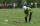15 boys and 10 girls are in the class. On school competition of them is selected 6-member team composed of 4 boys and 2 girls. How many ways can we select students?
• Boys and glasses2/3 of the students in Sarah’s class are boys. Of the boys, 1/3  of them wear glasses. What fraction of the students wear glasses?